ď»ż

### Introduzione

#### Abstract

En
Given a countably paracompact normal space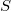$S$ with a closed subspace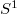$S^1$ and a finite derected graph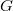$G$ with a subgraph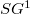$SG^1$, there are bijections from the sets of homotopy classes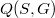$Q(S,G)$ and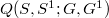$Q(S,S^1;G,G^1)$ to the sets of o*-homotopy classes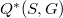$Q^\ast(S,G)$ and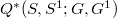$Q^\ast(S,S^1;G,G^1)$ respectively (see 3).Here we prove,by some examples,that certain conditions on$S$ weaker than the ones required in (3) are not sufficent to prove the results given above.

DOI Code: §

Full Text: PDFThis work is licensed under a Creative Commons Attribuzione - Non commerciale - Non opere derivate 3.0 Italia License.